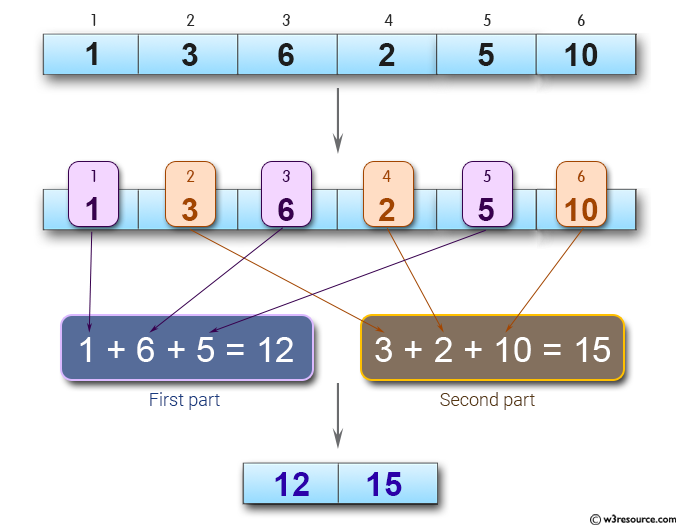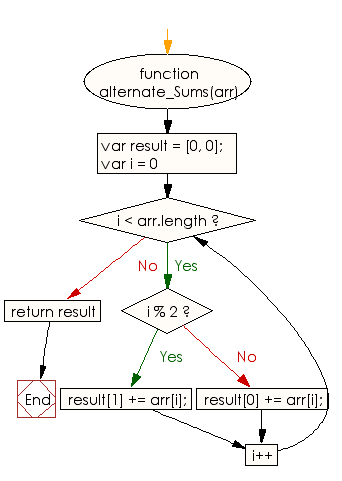# JavaScript: Compute the sum of two parts and store into an array of size two

## JavaScript Basic: Exercise-85 with Solution

Write a JavaScript code to divide a given array of positive integers into two parts. First element goes to first part, second element goes to second part, and third element goes to first part and so on. Now compute the sum of two parts and store into an array of size two.

Pictorial Presentation:Sample Solution:

HTML Code:

``````<!DOCTYPE html>
<html>
<meta charset="utf-8">
<meta name="viewport" content="width=device-width">
<title>Compute the sum of two parts and store into an array of size two</title>
<body>

</body>
</html>
```
```

JavaScript Code:

``````function alternate_Sums(arr) {
var result = [0, 0];
for(var i = 0; i < arr.length; i++)
{
if(i % 2) result += arr[i];
else
result += arr[i];
}
return result
}

console.log(alternate_Sums([1, 3, 6, 2, 5, 10]))
```
```

Sample Output:

```[12,15]
```

Flowchart:ES6 Version:

``````function alternate_Sums(arr) {
const result = [0, 0];
for(let i = 0; i < arr.length; i++)
{
if(i % 2) result += arr[i];
else
result += arr[i];
}
return result
}

console.log(alternate_Sums([1, 3, 6, 2, 5, 10]))
``````

Live Demo:

See the Pen javascript-basic-exercise-85 by w3resource (@w3resource) on CodePen.

What is the difficulty level of this exercise?

Test your Programming skills with w3resource's quiz.

﻿

## JavaScript: Tips of the Day

Converts a string to title case

Example:

```const toTitleCase = str =>
str
.match(/[A-Z]{2,}(?=[A-Z][a-z]+[0-9]*|\b)|[A-Z]?[a-z]+[0-9]*|[A-Z]|[0-9]+/g)
.map(x => x.charAt(0).toUpperCase() + x.slice(1))
.join(' ');
console.log(toTitleCase('some_database_field_name')); // 'Some Database Field Name'
console.log(toTitleCase('Some label that needs to be title-cased')); // 'Some Label That Needs To Be Title Cased'
console.log(toTitleCase('some-package-name')); // 'Some Package Name'
console.log(toTitleCase('some-mixed_string with spaces_underscores-and-hyphens')); // 'Some Mixed String With Spaces Underscores And Hyphens'
```

Output:

```"Some Database Field Name"
"Some Label That Needs To Be Title Cased"
"Some Package Name"
"Some Mixed String With Spaces Underscores And Hyphens"
```